# DataReconciliation¶

The objective of data reconciliation is to use physical models to decrease measurement uncertainties on physical quantities. Data reconciliation is possible only when redundant measurements are available for a given physical quantity.

## Defining DataReconciliation Problem in OpenModelica¶

To define DataReconciliation Problem in OpenModelica, The Modelica model must be defined with the following

• The list of variables of interest, which is defined in the modelica model as a special variable attribute (uncertain=Uncertainty.refine)

• The list of approximated equations. which is defined in the modelica model as a special annotation (__OpenModelica_ApproximatedEquation=true)

The list of Variable of interest are mandatory and the list of approximated equations are optional. An example of modelica model with dataReconciliation problem is given below,

```model Splitter1
Real Q1(uncertain=Uncertainty.refine);
Real Q2(uncertain=Uncertainty.refine);
Real Q3(uncertain=Uncertainty.refine);
parameter Real P01 =3;
parameter Real P02 =1;
parameter Real P03 =1;
Real T1_P1, T1_P2, T2_P1, T2_P2, T3_P1, T3_P2;
Real V_Q1, V_Q2, V_Q3;
Real T1_Q1, T1_Q2, T2_Q1, T2_Q2, T3_Q1, T3_Q2;
Real P, V_P1, V_P2, V_P3;
equation
T1_P1 = P01;
T2_P2 = P02;
T3_P2 = P03;
T1_P1 - T1_P2 = Q1^2 annotation (__OpenModelica_ApproximatedEquation=true);
T2_P1 - T2_P2 = Q2^2 annotation (__OpenModelica_ApproximatedEquation=true);
T3_P1 - T3_P2 = Q3^2 annotation (__OpenModelica_ApproximatedEquation=true);
V_Q1 = V_Q2 + V_Q3;
V_Q1 = T1_Q2;
T1_Q2 = Q1;
V_Q2 = T2_Q1;
T2_Q1 = Q2;
V_Q3 = T3_Q1;
T3_Q1 = Q3;
T1_P2 = V_P1;
V_P1 = P;
T2_P1 = V_P2;
V_P2 = P;
T3_P1 = V_P3;
V_P3 = P;
T1_Q1 = Q1;
T2_Q2 = Q2;
T3_Q2 = Q3;
end Splitter1;
```

After defining the modelica model, the users must define the dataReconciliation Input File.

### DataReconciliationInputFile¶

The dataReconciliation Input file is a csv file with the the following headers,

• Variable Names - names of the Uncertainty variables, given in the modelica model

• Measured Value-x – Values given by the users

• HalfWidthConfidenceInterval – Values given by the users, which computes Covariance Matrix Sx

• xi – co-relation- coefficients

• xk - co-relation- coefficients

• rx_ik- value associated with co-relation coefficients

The first 3 column, Variable Names, Measured Value-x and HalfWidthConfidenceInterval are mandatory The remaining column xi, xk, rx_ik are correlation-coefficients which are optional. An example csv file is given below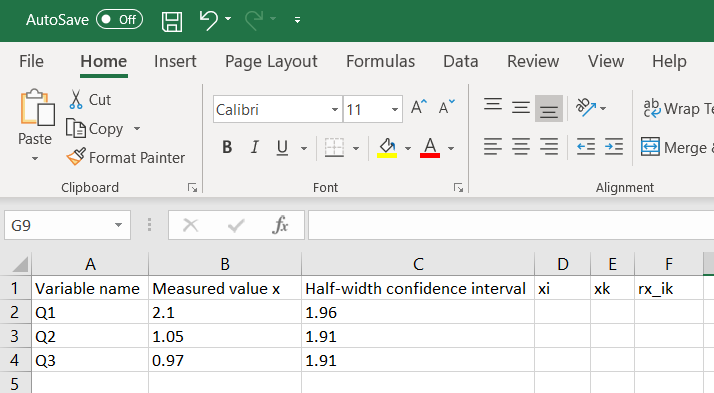Figure 142 An example DataReconciliationInput file(.csv)

The ordering of variables in the csv files should be defined in correct order on how it is declared in the model, for example in the above example we have uncertain variables defined in the following order Q1,Q2 and Q3 and the same order should be followed for the csv file in order to match the jacobian columns generated for dataReconciliation Otherwise the dataReconciliation procedure computes wrong results.

Now we are ready to run the DataReconciliation procedure in OpenModelica.

## DataReconcilation Support with Scripting Interface¶

The data Reconciliation procedure is possible to run through OpenModelica scripting interface(.mos file). An example mos script (a.mos) is present below.

```setCommandLineOptions("--preOptModules+=dataReconciliation");
getErrorString();
getErrorString();
simulate(DataReconciliationTests.Splitter1,simflags="-reconcile -sx=./Splitter1_Sx.csv -eps=0.0023 -lv=LOG_JAC");
getErrorString();
```

To start the dataReconciliation procedure via command line interface, the users have to enable the dataReconciliation module which is done via setCommandLineOptions("--preOptModules+=dataReconciliation") which runs the extraction algorithm for dataReconciliation procedure. And finally the users must specify 3 runtime simulation flags given below

1. reconcile – runtime flag which starts the dataReconciliation Procedure

2. sx – csv file Input

3. eps – small value given by users

The Flag -lv=LOG_JAC is optional and can be used for debugging.

And finally run the mos script(a.mos) with omc

>> omc a.mos

The HTML Reports, the Csv files and the debugging log are generated in the current directory see DataReconcilation Results.

## DataReconciliation Support in OMEdit¶

The DataReconciliation setup can be launched by,

• Selecting Simulation > Simulation Setup from the menu. (requires a model to be active in ModelWidget)

• Clicking on the Simulation Setup toolbar button. (requires a model to be active in ModelWidget)

• Right clicking the model from the Libraries Browser and choosing Simulation Setup.

### TranslationFlag Tab¶

From the translationFlag tab, do the following,

• check the Enable dataReconciliation checkbox.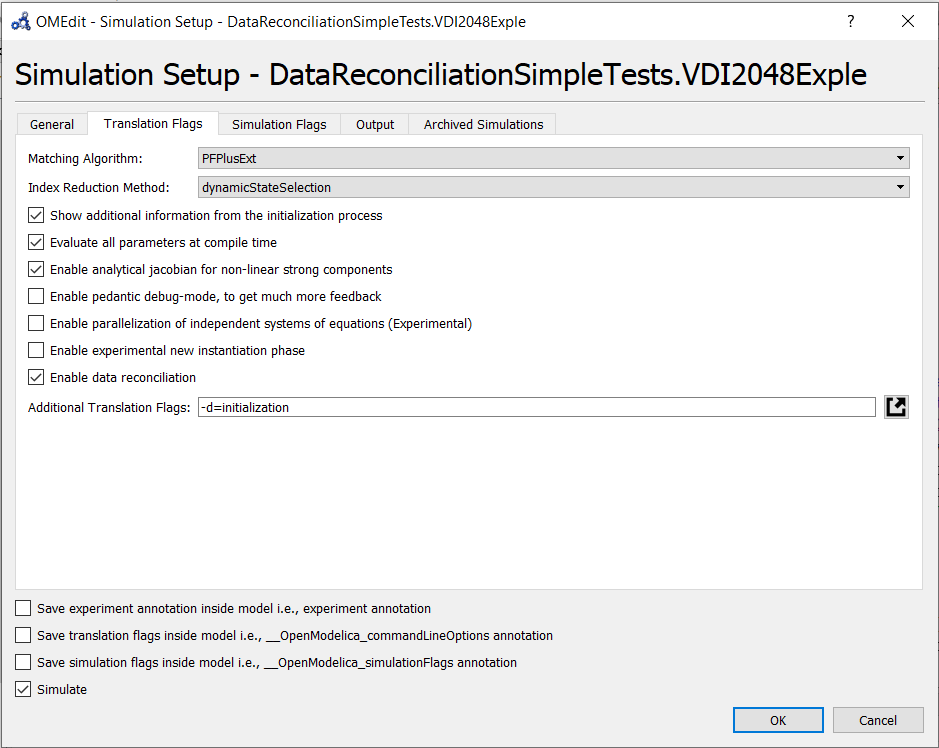Figure 143 Setting DataReconciliation TraslationFlag

### SimulationFlag Tab¶

From the SimulationFlag tab, do the following,

• check the DataReconciliation Algorithm for Constrained Equation checkbox.

• load the input file with dataReconciliation inputs, only csv file is accepted.

• fill in the Epsilon value (e.g) 0.001

And finally press the ok button to start the dataReconciliation procedure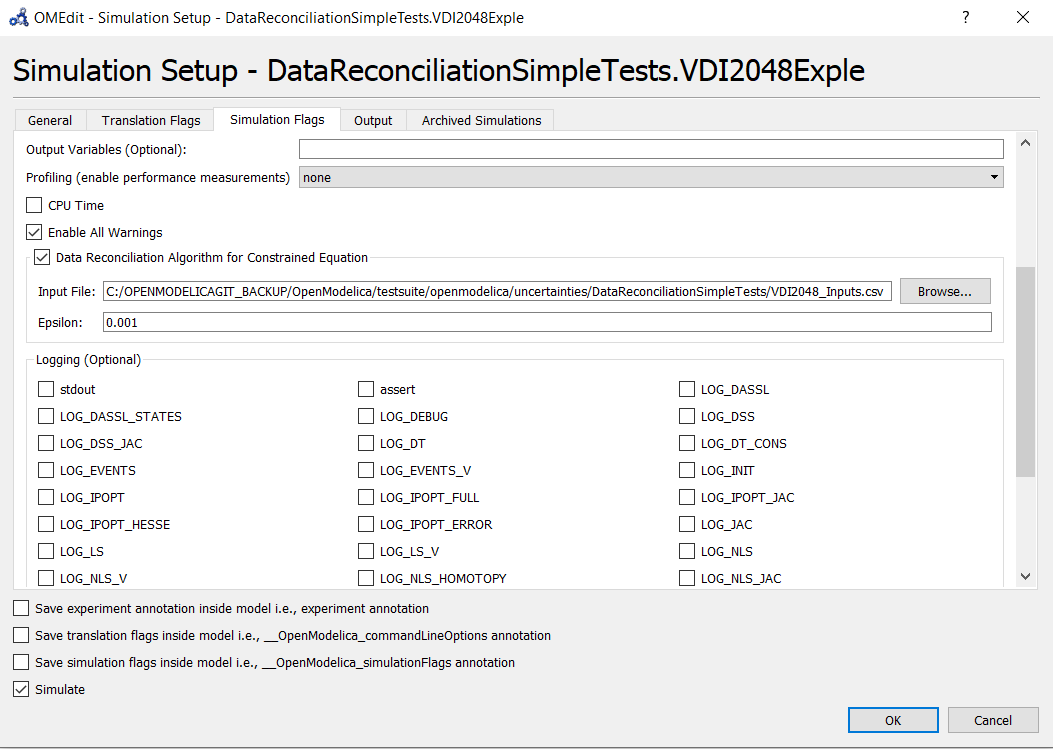Figure 144 Setting DataReconciliation SimuationFlag

### Generating the InputFile and Running the DataReconciliation¶

Generating an empty csv file with variable names makes it easy for the users to fill in the datas, so that ordering of variables and names are not mismatched. This is an important step as variable ordering should match with the jacobian columns generated for dataReconciliation procedure. The input file is named as “modelname_Inputs.csv” which is generated in the current working directory of the model. This step shall be done for the first time and the next time when running the dataReconciliation for the same model, we can directly set the input file and run the DataReconciliation procedure.

This is done in 2 steps.

And then from the plotting window variable browser, right click on the model and select the “re-simulate Setup” as shown below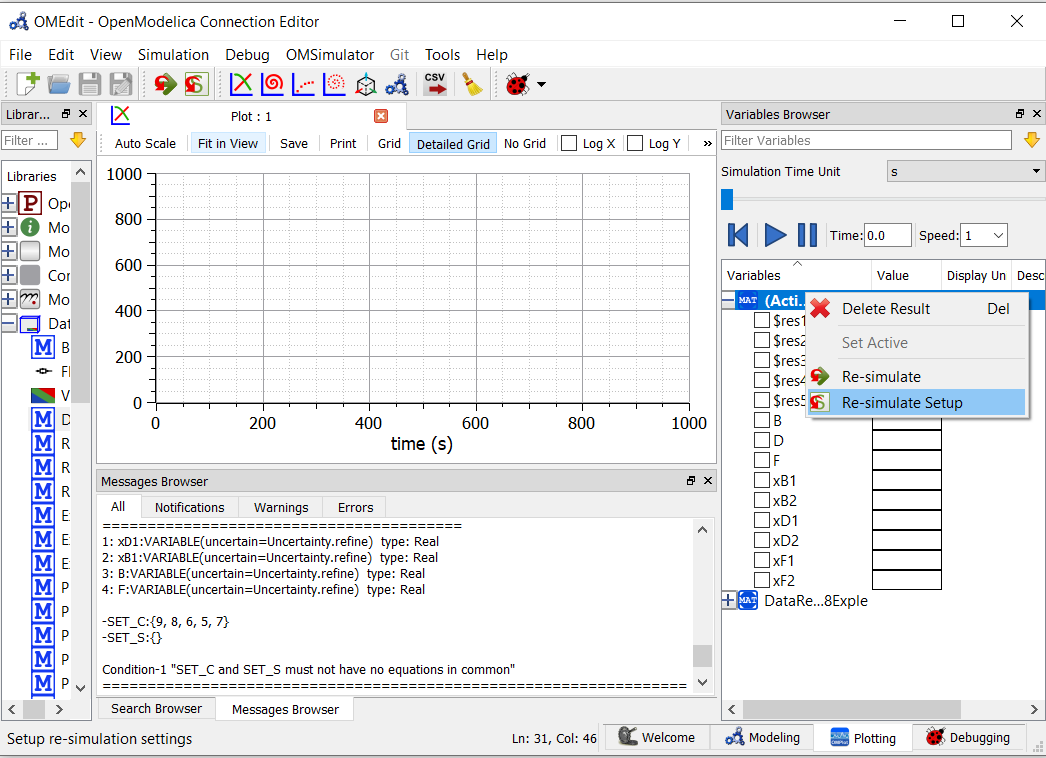Figure 145 Select the re-simulate setup

Which opens the simulation set-up dialog window and select the simulation Flag tab defined in SimulationFlag Tab. and load the csv file and fill in the epsilon value and press the “Ok” button to start the Data Reconciliation Procedure.

## DataReconcilation Results¶

After the Data Reconciliation procedure is completed, the results are generated in the working directory. The default working directory in OMEdit is set to local temp directory of the operating system. The users can change the working directory of OMEdit by, Tools > Options > General > WorkingDirectory

A separate working directory is created in the working directory. The directory is named based on the modelName and the result files are stored in that directory. Two result files are generated namely.

• HTML Report.

• CSV file

An Example of Result directory is given below,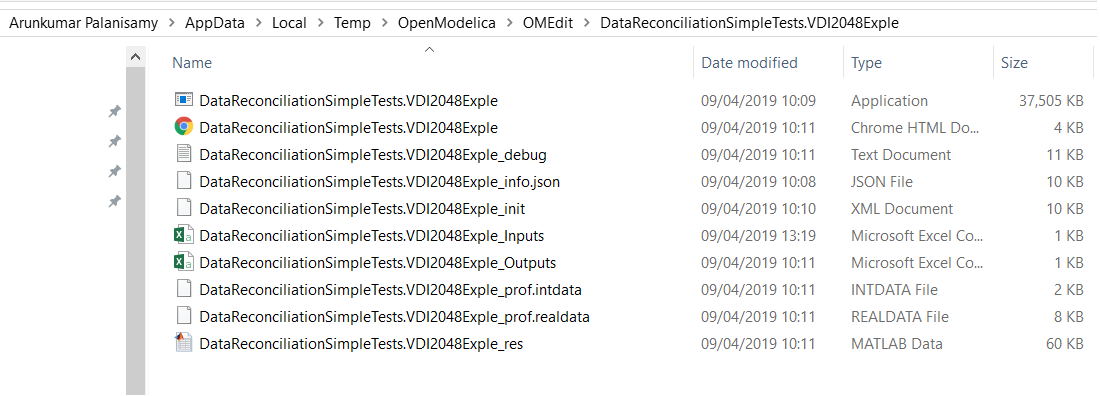Figure 146 Result Directory Structure

### HTML Report¶

The html report is named with modelname.html. The Html report contains 3 section namely 1. Overview 2. Analysis and 3. Results

The Overview section provides the general details of the model such as Modelicafile, ModelName, ModelDirectory, InputFiles and Generated Date and Time of the Report.The Analysis section provides information about the data Reconciliation procedure such as Number of Extracted equations in setC, Number of variable to be Reconciled which are Variable of interest, Number of Iterations to Converge, Final Converged Value ,Epsilon value provided by the users and Results of Global test.

The Results section provides the numerical values computed by the data Reconciliation algorithm. The table contains 8 columns namely,

1. Variables to be Reconciled – names of the Uncertainty variables, given in the modelica model

2. Initial Measured Values – numerical values given by the users

3. Reconciled Values – Calculated values according to Data Reconciliation Procedure.

4. Initial Uncertainty Values – Half Width confidence interval provides by the users, which is later used to compute the Covariance Matrix Sx.

5. Reconciled Uncertainty Values – Calculated Values according to Data Reconciliation Procedure.

6. Results of Local Tests – Calculated values according to Data Reconciliation Procedure

7. Values of Local Tests – Calculated values according to Data Reconciliation Procedure

8. Margin to correctness – Calculated values according to Data Reconciliation Procedure

A sample HTML Report generated for Splitter1.mo model is presented below.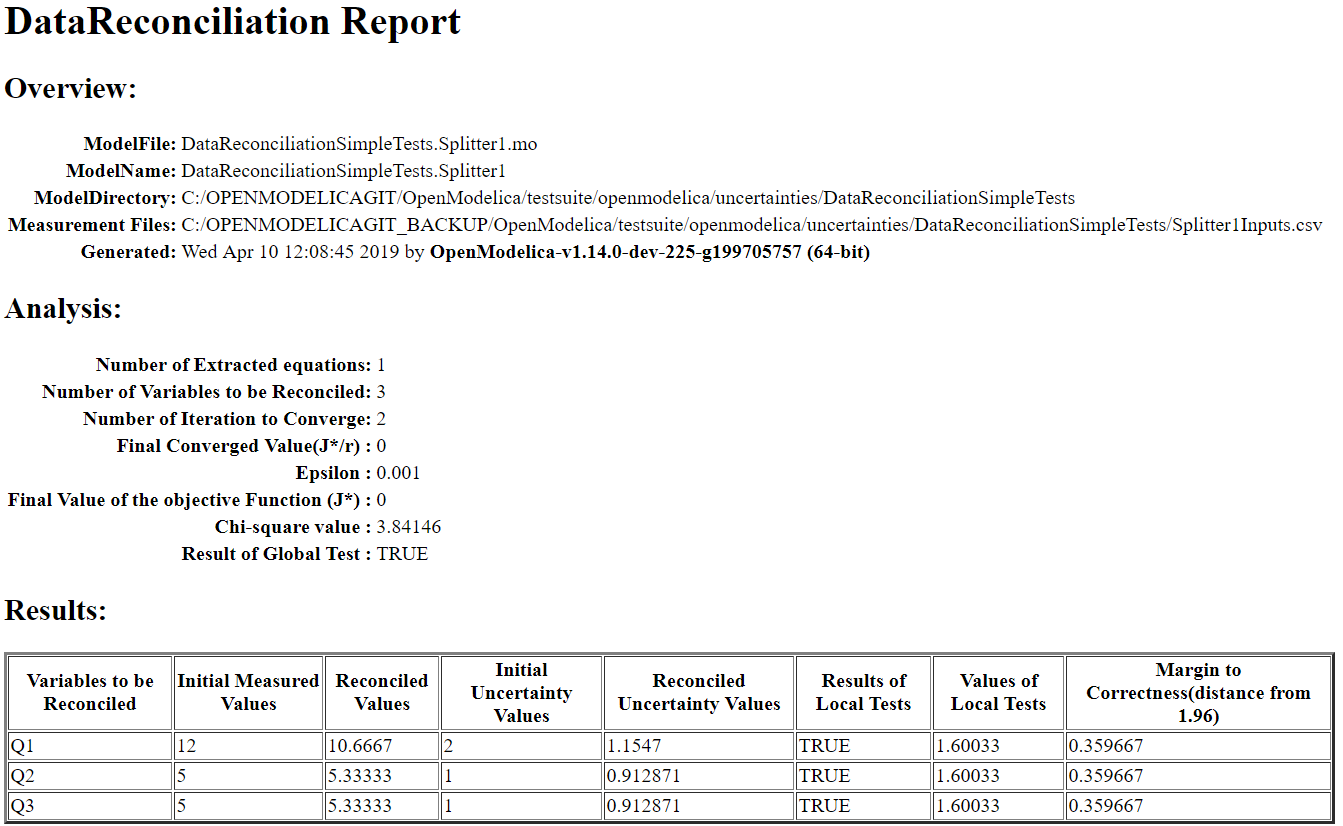Figure 147 HTML Report

### Csv file¶

Along with the Html Report, an output csv file is also generated which mainly contains the Results section of the HTMl report in a csv format. The csv file is named with modelname_Outputs.csv. An example output csv file is presented below.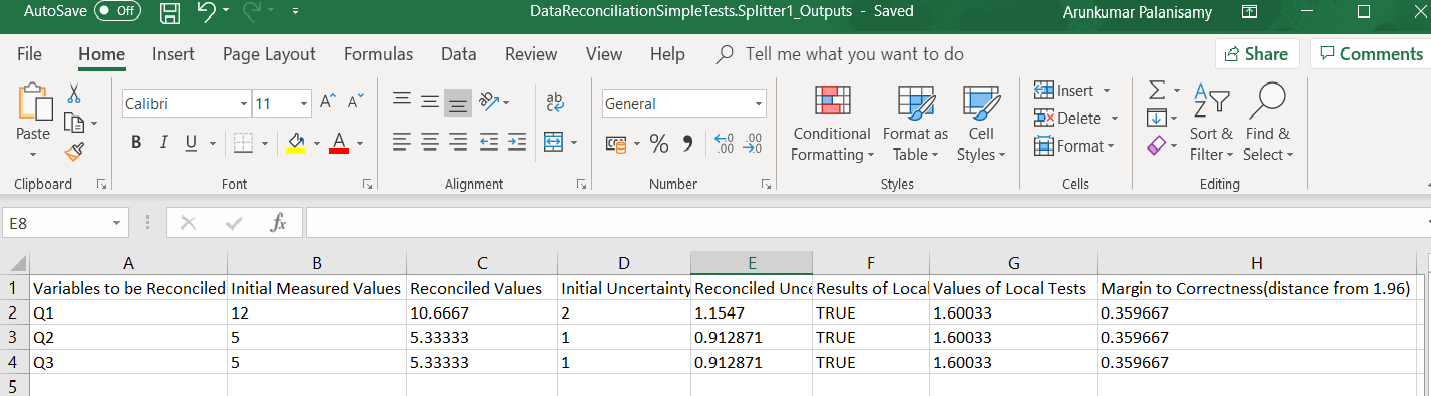Figure 148 Output Csv file

### Logging and Debugging¶

All the Computations of data Reconciliation procedure are logged into log file. The log file is named as modelname_debug.log. For Detailed Debugging the flag LOG_JAC checkbox can be checked see SimulationFlag Tab.